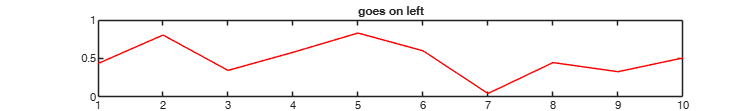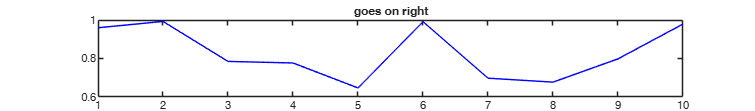# upperfracpos

upperfracpos positions two figures on the upper part of the screen.

## Syntax

• upperfracpos(hfigl , hfigr , fraction)example

## Description

 upperfracpos(hfigl , hfigr , fraction) Example of use of upperfracpos.

## Examples

expand all

###Example of use of upperfracpos.

close all;
% create two figures, rescale and position them
hfigl = figure; plot(sin(rand(10,1)),'r'); title('goes on left');
hfigr = figure; plot(cos(rand(10,1)),'b'); title('goes on right');
upperfracpos(hfigl , hfigr , 0.5);
% now rescale the figures to a smaller proportion
upperfracpos(hfigl , hfigr , 0.2);
% this is just to bring the rescaled figures in the screen foreground
figure(hfigl); figure(hfigr);## Input Arguments

### hfigl — handle for figure. matlab.ui.Figure.

Graphics handle of the figure which will be put on the upper left part of the screen

Data Types: single| double

### hfigr — handle for figure. matlab.ui.Figure.

Graphics handle of the figure which will be put on the upper right part of the screen

Data Types: single| double

### fraction — fraction to occupy. Scalar between 0 and 1.

Fraction of the screen which will be occupied.

Data Types: single| double

## Output Arguments

Tufte E.R. (1983), "The visual display of quantitative information", Graphics Press, Cheshire.